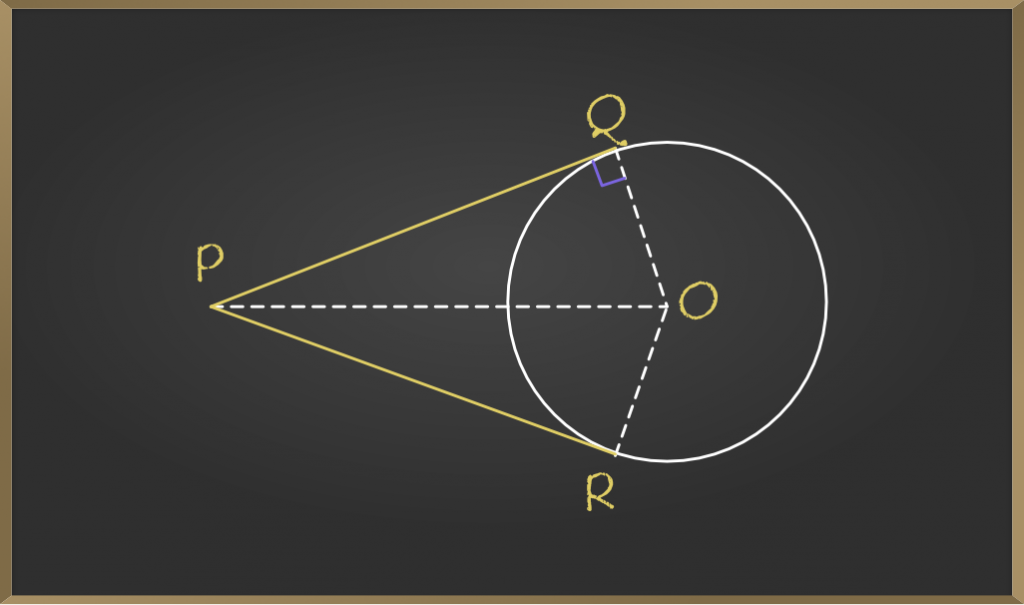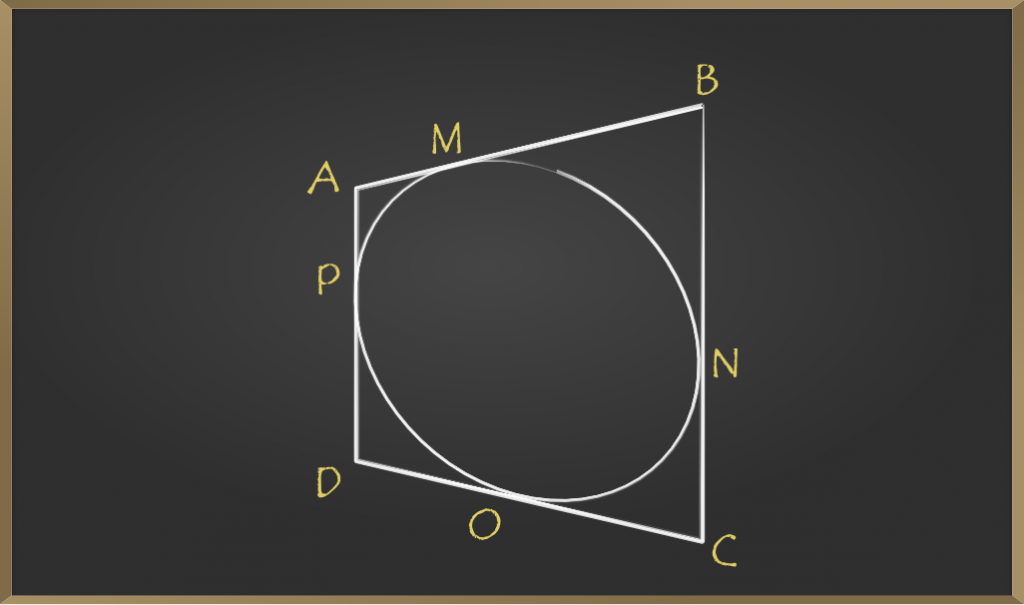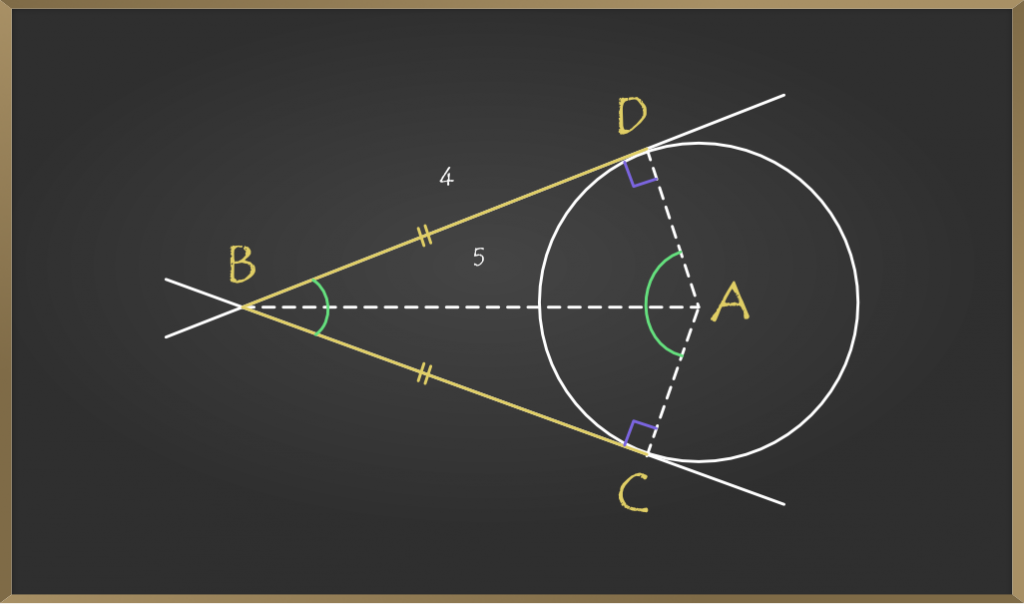GeeksforGeeks App
Open AppBrowser
Continue

# Theorem – The lengths of tangents drawn from an external point to a circle are equal – Circles | Class 10 Maths

Tangent is a straight line drawn from an external point that touches a circle at exactly one point on the circumference of the circle. There can be an infinite number of tangents of a circle. These tangents follow certain properties that can be used as identities to perform mathematical computations on circles.

Here, in this article, we will learn about one of such properties i.e. the tangents drawn from an external point to a circle are of equal length.

### To Prove: The lengths of tangents drawn from an external point to a circle are equal

Let PQ and PR be the two tangents drawn to the circle of Centre O as shown in the figure.### Construction

Draw a line segment, from Centre O to external point P { i.e. P is the intersecting point of both the tangents}

Now ∆POR and ∆POQ

In order to prove they have the same length, we will first prove that both triangles are similar.

We know that the tangents make a right angle with a radius of the circle.

Here, OR and OQ is the radius of the circle

So, ∠OQP = ∠ORP = 90°
Now, it is clear that both the triangles ∆POQ and ∆POR are right-angled triangles and a common hypotenuse OP in them.

### Proof

Now proving the similarity between triangles ∆POQ and ∆POR

Here,

∠PQO = ∠PRO = 90°
Common hypotenuse OP between them.

And OQ = OR [Radius of circle].
So, by the R.H.S. rule of similarity

∆POQ ~ ∆POR

Hence, both the triangles are similar to each other.

Therefore,
OP/OP = PQ/PR = OQ/OR

PQ/PR = 1 {since OP/OP = 1} ;

Hence, PQ = PR;

Hence, Proved that The lengths of tangents drawn from an external point to a circle are equal.

### Sample Problems based on the Theorem

Problem 1: Two tangents are drawn from an external point on a circle of area 3 cm. Find the area of the quadrilateral formed by the two radii of the circle and two tangents if the distance between the centre of the circle and the external point is 5 cm.Solution:

It is taken note that if PO is joined, then ΔPQO will be right-angled at Q, and so the Pythagoras Theorem applies:

Given that :
OQ = 3 cm
OP = 5 cm
Using Pythagoras we can find the OP:
OP^2 = OQ^2 + PQ^2
25 = 9 + QP^2
Qp = 4 cm.

Since both, the tangents have the same length and also we know that the triangles are congruent hence, Both triangles would have the same Area.

Therefore,

Total Area = 2 times the area of the triangle
Area = 2 * (1/2) * 4 * 3
Area = 12 cm^2

Hence the total Area is 12 cm^2.

Problem 2: How many tangents can be drawn to circle S from a point P inside circle S? Explain your findings.

Solution:

The answer is none. But the important part here is the explanation.
Suppose If PA is a tangent to Circle S from an internal point P, then the points P, O and A will form a right-angled triangle with hypotenuse OP.
We know that OA is a radius of circle S, since P is inside S, OP must be less than OA ( from the rule of the hypotenuse in a right-angled triangle). Thus, the above-assumed triangle cannot exist.
Hence, proved that no tangent can be drawn from an interior point P to circle S.

Problem 3: A circle is inscribed in the quadrilateral ABCD, prove that AB + CD = AD + BC.
The figure is given for your reference.Solution:

We Already know that the length of Tangents drawn from point A will be the same.
From this, we infer that,
AP = AM —(1)

Similarly, for tangents drawn from point B,
BN = BM —(2)

In the same manner for the Tangents drawn from point C,
CN = CO —(3)

In the same manner for the Tangents drawn from point D,
DP = DO —(4)

We have :
AM + BM + CO + DO = AP + BN + CN + DP

Now;
⇒ AP + PD + BN + NC = AM + MB + DO + OC
⇒ AD + BC = AB + CD

Hence proved.

Problem 4: From an external point B, tangents BC and BD are drawn to a circle with centre A so that the length of each tangent is 4 cm, and AB = 5 cm. What is the radius of the circle?Solution:

It is taken note that if AB is joined, then ΔABC will be right-angled at C, and so the Pythagoras Theorem applies:

AB2 = AC2 + BC2
AC2 = AB2 – BC2 = 25 – 16 = 9

Hence, AC = 3

My Personal Notes arrow_drop_up
Related Tutorials